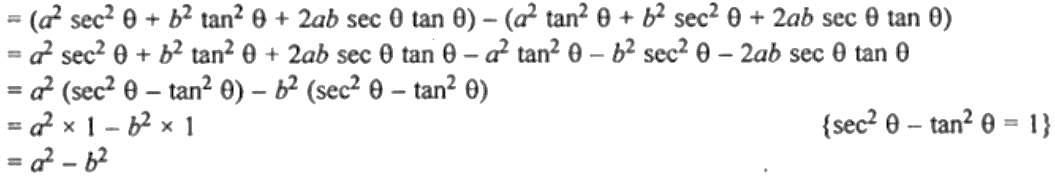Guru

# If x – a sec θ + b tan θ and y = a tan θ + b sec θ, prove that x2 – y2 = a2 – b2.

• 0

This is an important question from the
Book- ML Aggarwal
Board- ICSE
Publication- Avichal
Chapter- Trigonometric Identities
Chapter number-18

If x – a sec θ + b tan θ and y = a tan θ + b sec θ, prove that x2 – y2 = a2 – b2.

Trigonometric Identities, class 10th, chapter 18th, question no34 , ICSE board, ML aggarwal..

Share

1. Given,

x =
a sec θ + b tan θ,

y = a tan θ + b sec θ

Now,

x2 – y2 = (a sec θ + b tan θ)2 – (a tan θ + b sec θ)2– Hence proved.

• 0